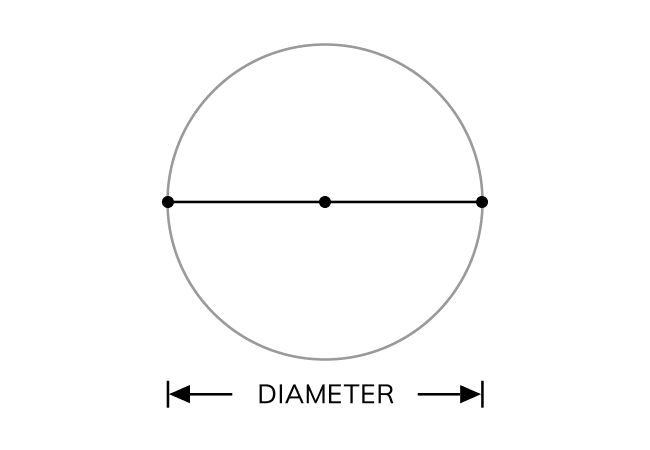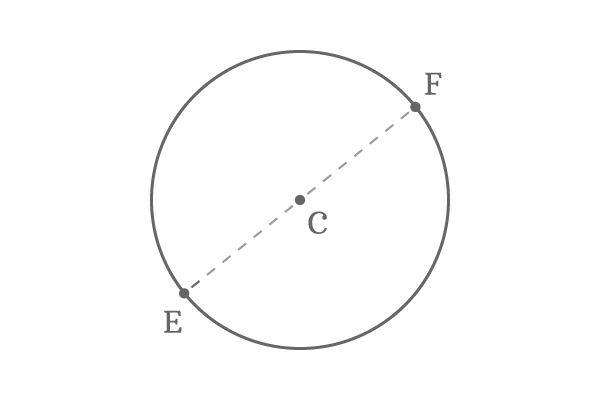Diameter

Definition

The straight distance between any two opposite points on the circumference through centre of the circle is called the diameter of the circle.Diameter is a distance between two points on the circumference but one point should be opposite to another point through the centre of the circle.

The diameter of a circle is twice the radius of the same circle geometrically and it is usually denoted by the letter $d$.

$Diameter \, (d) = 2 \times radius$

This formula is used to find the diameter of a circle from the radius of the circle in mathematics.

Example$E$ and $F$ are two opposite points on the circumference of the circle in the point of view of its centre ($C$).

The straight distance from point $E$ to point $F$ through centre $C$ is known as the diameter of the circle. The straight path between points $E$ and $F$ seems the length of a line segment $\overline{EF}$.

Diameter ($d$) $= EF$

In this case, the distance between points $C$ and $E$, also distance between points $C$ and $F$ is radius of the circle.

$d = 2 \times CF$ (or) $d = 2 \times CE$

Latest Math Topics
Latest Math Problems
Email subscription
Math Doubts is a best place to learn mathematics and from basics to advanced scientific level for students, teachers and researchers. Know more